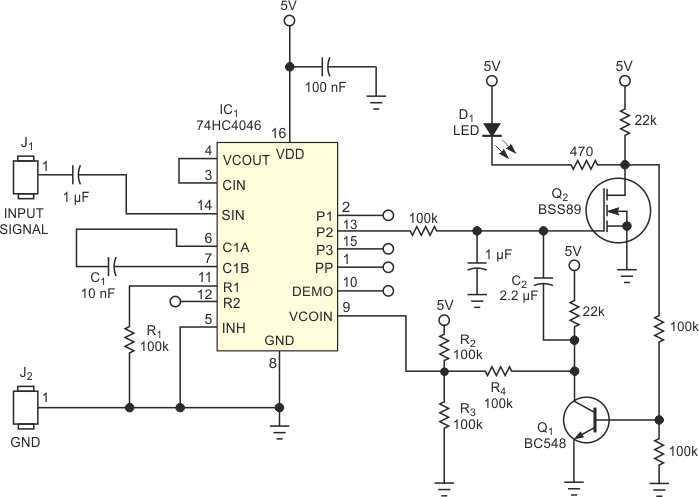# Frequency comparator has status output

The original application for the circuit in Figure 1 was to check the number of revolutions of an engine with only one LED as an indicator. The measurement of the number of revolutions usually involves sensors with a frequency output proportional to the number of revolutions. The circuit compares the frequency output of such a sensor with a lower and upper limit and gives a visual result, using one LED. If the frequency is below the lower limit, the LED remains unlit. If the frequency is between the limits, the LED blinks at a constant rate, and if the speed is higher than the upper limit, the LED stays permanently lit. Although a microcontroller can do this job, it is sometimes better to use an analog circuit – for example, if the frequency you want to check is too high for a simple controller. The circuit in Figure 1 uses one standard, inexpensive IC, and you need not write any software. It is also less costly than a comparable microcontroller-based circuit.Figure 1. This analog frequency comparator uses an LED to indicate upper and lower frequency limits.

The main part is IC1, a 74HC4046 PLL chip. With a 12 V supply, you can also use the CD4046 without an additional voltage regulator. The chip contains an oscillator, a phase comparator, and one amplifier for the input signal. The input signal connects to the input of the phase comparator with its integrated ac amplifier. The circuit compares this amplified signal with the VCO frequency. This frequency is adjustable, using C1, R1, and the voltage on Pin 9. If the frequency of the input signal is lower than that of the VCO, the output of the phase comparator (Pin 13) is low. In this case, Q2 is off, Q1 is on, and the LED is off, indicating “low frequency.” Resistors R2, R3, and R4 determine the voltage on Pin 9 and the lower frequency limit switching point. If the frequency of the input signal increases and reaches the value of the VCO's frequency, the phase comparator's output switches high.

This high-level output turns Q2 on and Q1 off. With Q1 off, the voltage of the VCO increases to a second higher value determined by R2 and R3, and the VCO generates the frequency for the high-limit switching point. If the frequency of the input signal is between these two limits, the phase comparator generates a rectangular waveform. The feedback capacitor, C2 determines the frequency of this waveform. With a value of 2.2 µF for C2, you can achieve a frequency of approximately 1 Hz. This frequency is the blinking rate for the LED. If the frequency of the input signal increases to a point higher than the upper VCO frequency value, the phase comparator output stays high, and the LED turns on permanently. With the values shown in Figure 1, the lower and upper frequency limits are 3.81 and 7.35 kHz, respectively.

## Materials on the topic

EDN